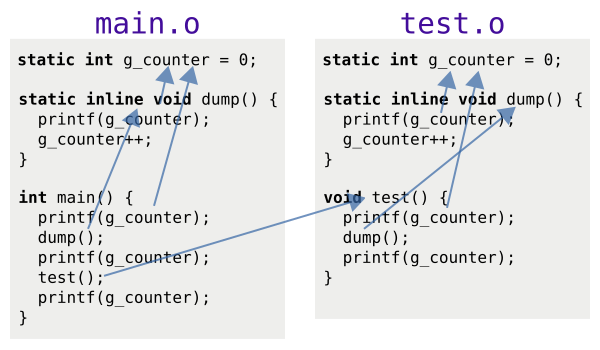# C++ 17 Inline Variable

Inline Variable（內嵌變數）是 C++ 17 新增的功能。Inline Variable 與 Inline Function（內嵌函式）相似，能讓我們在多個 Translation Unit（編譯單元）定義同樣的變數。鏈結器（Linker）在處理多個 Object File（目的檔）的時候會再將名字相同的 Inline Variable 合併為一個變數。

1. 動機 -- 介紹 C++ 標準新增 Inline Variable 的動機。
2. Inline Variable -- 介紹 Inline Variable 的語法與注意事項。
3. 替代方案 -- 介紹 C++ 17 以前 Inline Variable 的替代選項。

## 動機

### 情境一：在標頭檔定義變數

situation1/example.h

```#ifndef EXAMPLE_H_
#define EXAMPLE_H_

extern int x;  // #1

extern void print();

#endif  // EXAMPLE_H_
```

situation1/example.cpp

```#include "example.h"
#include <iostream>

int x = 42;  // #2

void print() {
std::cout << x << std::endl;
}
```

```#ifndef EXAMPLE_H_
#define EXAMPLE_H_

int x;  // #3

extern void print();

#endif  // EXAMPLE_H_
```

```#include "example.h"
#include <iostream>

void print() {
std::cout << x << std::endl;
}
```

```#include "example.h"

int main() {
print();
}
```

```\$ g++ example.cpp main.cpp
/tmp/cc68uH4D.o:(.data+0x0): multiple definition of 'x'
/tmp/cc0QdmVR.o:(.data+0x0): first defined here
collect2: error: ld returned 1 exit status
```

### 情境二：常數靜態資料成員

situation2/static_const_example.h

```#ifndef STATIC_CONST_EXAMPLE_H_
#define STATIC_CONST_EXAMPLE_H_

class Example {
public:
static const int x = 42;  // #4
};

#endif  // STATIC_CONST_EXAMPLE_H_
```

situation2/main.cpp

```#include "static_const_example.h"
#include <iostream>

int main() {
std::cout << Example::x << std::endl;
}
```

```\$ g++ main.cpp
\$ ./a.out
42
```

situation2_odr/main_odr.cpp

```#include "static_const_example.h"
#include <iostream>

int main() {
std::cout << &Example::x << std::endl;  // modified
}
```

```\$ g++ main_odr.cpp
/tmp/cc7zHlwx.o: In function 'main':
main_odr.cpp:(.text+0x7): undefined reference to 'Example::x'
collect2: error: ld returned 1 exit status
```

situation2_odr/static_const_example.cpp

```#include "static_const_example.h"

const int Example::x;
```

```\$ g++ static_const_example.cpp main.cpp
\$ ./a.out
0x55e3ea5089b8
```

Note

## Inline Variable

Inline Variable（內嵌變數）是以 `inline` 關鍵字修飾的變數（包含全域變數或靜態資料成員）（`#5``#6``#8``#10`）；以 `constexpr` 關鍵字修飾的靜態資料成員也是 Inline Variable（`#9`）：

```inline int global_variable = 42;               // #5

class Example {
public:
static inline int data_member_1{42};         // #6
static int data_member_2;                    // #7
static inline const int data_member_3 = 42;  // #8

static constexpr int data_member_4 = 42;     // #9
};

inline int Example::data_member_2 = 42;        // #10
```

`inline` 關鍵字修飾的靜態資料成員能在「類別定義（Class Definition）」內直接以「等號（`#8``#9`）」或「大刮號（`#6`）」初始化。如果不想要直接在類別定義內初始化，則應該在定義靜態資料成員的時候加上 `inline` 關鍵字（`#7``#10`）。

Inline Variable（內嵌變數）與 Inline Function（內嵌函式）或 Template（樣版）相似。只要 Translation Unit 有使用到該 Inline Variable，編譯器產生的 Object File 就會包含一份完整的定義。然而編譯器會將 Inline Variable 標上特別的記號，當鏈結器看到該記號就會將同名的 Inline Variable 再合併為一個變數。這也保證每一個有 External Linkage 的 Inline Variable 都只會有一個位址。

### 範例

counter.h

```#ifndef COUNTER_H_
#define COUNTER_H_

#include <stdio.h>

inline int g_counter = 0;

inline void dump() {
printf("dump: value=%d ptr=%p\n", g_counter, &g_counter);
g_counter++;
}

#endif  // COUNTER_H_
```

main.cpp

```#include "counter.h"

extern void test();

int main() {
printf("main: value=%d ptr=%p\n", g_counter, &g_counter);
dump();
printf("main: value=%d ptr=%p\n", g_counter, &g_counter);
test();
printf("main: value=%d ptr=%p\n", g_counter, &g_counter);
}
```

test.cpp

```#include "counter.h"

void test() {
printf("test: value=%d ptr=%p\n", g_counter, &g_counter);
dump();
printf("test: value=%d ptr=%p\n", g_counter, &g_counter);
}
```

```\$ g++ main.cpp test.cpp -std=c++17

\$ ./a.out
main: value=0 ptr=0x55b821476014
dump: value=0 ptr=0x55b821476014
main: value=1 ptr=0x55b821476014
test: value=1 ptr=0x55b821476014
dump: value=1 ptr=0x55b821476014
test: value=2 ptr=0x55b821476014
main: value=2 ptr=0x55b821476014
``````\$ g++ main.cpp test.cpp -std=c++17

\$ ./a.out
main: value=0 ptr=0x5609714ab014
dump: value=0 ptr=0x5609714ab014
main: value=1 ptr=0x5609714ab014
test: value=0 ptr=0x5609714ab018  # different
dump: value=1 ptr=0x5609714ab014
test: value=0 ptr=0x5609714ab018  # different
main: value=2 ptr=0x5609714ab014
``````\$ g++ main.cpp test.cpp -std=c++17

\$ ./a.out
main: value=0 ptr=0x5574838ca014
dump: value=0 ptr=0x5574838ca014
main: value=1 ptr=0x5574838ca014
test: value=0 ptr=0x5574838ca018  # different
dump: value=0 ptr=0x5574838ca018  # different
test: value=1 ptr=0x5574838ca018  # different
main: value=1 ptr=0x5574838ca014
```### 注意事項：初始化順序

init_order/example.h

```#ifndef EXAMPLE_H_
#define EXAMPLE_H_

#include <stdio.h>

class Example {
public:
Example(const char *name) {
printf("Example(this=%p, name=\"%s\")\n", this, name);
}
};

#endif  // EXAMPLE_H_
```

init_order/main.cpp

```#include "example.h"

Example main0("main0");

inline Example b("b");
inline Example a("a");

Example main1("main1");

int main() {
printf("main0=%p\n", &main0);
printf("b=%p\n", &b);
printf("a=%p\n", &a);
printf("main1=%p\n", &main1);
}
```

init_order/a.cpp

```#include "example.h"

inline Example a("a");
```

init_order/b.cpp

```#include "example.h"

inline Example b("b");
```

```\$ g++ main.cpp -std=c++17

\$ ./a.out
Example(this=0x56540ceb5011, name="main0")
Example(this=0x56540ceb5013, name="b")
Example(this=0x56540ceb5014, name="a")
Example(this=0x56540ceb5012, name="main1")
...
```

```\$ g++ a.cpp b.cpp main.cpp -std=c++17; ./a.out
Example(this=0x55bae2207011, name="a")
Example(this=0x55bae2207020, name="b")
Example(this=0x55bae2207030, name="main0")
Example(this=0x55bae2207031, name="main1")
...

\$ g++ b.cpp a.cpp main.cpp -std=c++17; ./a.out
Example(this=0x5579258f4011, name="b")
Example(this=0x5579258f4020, name="a")
Example(this=0x5579258f4030, name="main0")
Example(this=0x5579258f4031, name="main1")
...

\$ g++ main.cpp a.cpp b.cpp -std=c++17; ./a.out
Example(this=0x56138e0cd011, name="main0")
Example(this=0x56138e0cd013, name="b")
Example(this=0x56138e0cd014, name="a")
Example(this=0x56138e0cd012, name="main1")
...
```

## 替代方案

### 內嵌函式加上靜態區域變數

```inline int &example() {
static int variable = 42;
return variable;
}
```

1. 如果 Static Local Variable 的型別是有建構式的類別，其建構式會在第一次執行 Inline Function 的時候被呼叫。視不同使用情境，這可能是優點也可能是缺點。
2. 在 C++ 98 這個方法不是 Thread-Safe。如果二個執行緒同時呼叫此 Inline Function，初始化 Static Local Variable 的過程可能會有 Data Race。

### 類別樣版與靜態資料成員

```template <typename T>
class Example {
public:
static int variable;
};

template <typename T>
int Example<T>::variable = 42;
```

## 參考資料

• P0386R2: Inline Variables
• N4659: Working Draft, Standard for Programming Language C++ (C++ 17 Draft); Section 10.1.6. The inline specifier [dcl.inline]; Section 6.6.3. Dynamic initialization of non-local variables [basic.start.dynamic]
• Using the GNU Compiler Collection (GCC), Vague Linkage

  Translation Unit（編譯單元）是指作為編譯器輸入的完整 C++ 原始碼（包含 `#include` 引入的程式碼）。C++ 編譯器會把一個 Translation Unit 編譯為一個 Object File（目的檔）。
  在常見的目標平台 GCC 會以 COMDAT 記錄 Inline Function、Inline Variable 與 Template Instantiation。如果目標平台不支援 COMDAT，GCC 編譯器會生成 Weak Symbol。
  C++ 標準只要求全域 Inline Variable 的初始化時間點可以是「先於 `main` 函式」或者「第一次使用（ODR-Use）該 Inline Variable 之前」。至於是那一個則是由 C++ 編譯器實作者決定。GCC 是直接在 `main` 函式之前初始化。

Note